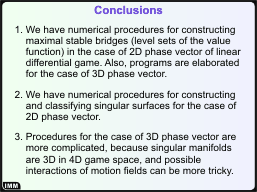# Numerical construction of singular surfaces in linear differential games

## Slide 1. Title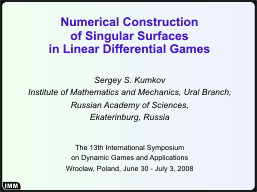My talk is devoted to an algorithm for constructing singular surfaces in some class of differential games.

## Slide 2. Singular SurfacesThe concept of singular surfaces in differential games was introduced by R.Isaacs in his famous book in 1965.

Singular surfaces can be defined as the surfaces in the game space where the bundles of optimal motions of the system have some peculiarities: fractures, splits, junctions. Investigation of singular surfaces is important because they give some "skeleton" of the game formation.

## Slide 3. Linear Differential Games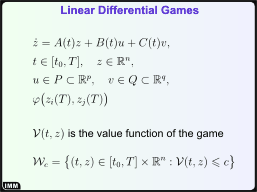The algorithm is suggested for differential games with the dynamics linear both on the phase variable and players' controls, fixed terminal time, geometric constraints for the players' controls and quasi-convex payoff function depending only on two components of the phase vector at the terminal instant. (A function is called quasi-convex if it has convex level sets, i.e., Lebesgue sets.) The constraints P and Q for the players' controls are convex compacta.

The main element of the solution of a differential game is its value function, that is, the function mapping a position in a game space to the optimal result of the game for this position. A convenient representaion of the value function is as a collection of its level sets (Lebesgue sets).

## Slide 4. Equivalent Game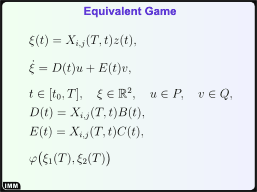All constructions are made in the framework of so-called equivalent game. Corresponding variable change is provided by a matrix Xi,j(T,t) combined of two rows (corresponding to the components of the phase vector, which define the payoff function) of the fundamental Cauchy matrix X(T,t) of the system dz/dt = A(t)z.

After the change, the time interval of the game, the constraints for the players' controls, and the payoff function stay the same. But now the payoff depends only on two components of the new equivalent phase vector.

The new game has two advantages. The first one is that the right-hand side of the dynamics does not depend of the new phase variable. The second one is that the new phase variable is two-dimensional. Therefore, the game space time × phase vector is three-dimensional, and the game is easier to study.

## Slide 5. Maximal Stable Bridges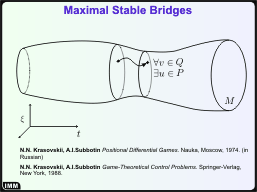In the taken class of the differential games, the level sets of the value function coincide with the maximal stable bridges. The concept of stable bridge was introduced in the book by N.N.Krasovskii and A.I.Subbotin in 1974 (in Russian; later, in 1988, there was a translation into English of its revised version).

A set is called stable if for any initial position inside the set and for any second player's control the first player can choose its control such that the resulting motions stays inside the set up to the terminal instant.

## Slide 6. Backward Procedure for Constructing Maximal Stable Bridges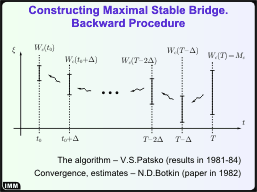In the beginning of 1980's in the Institute of Mathematics and Mechanics (Ekaterinburg, Russia), some algorithms and corresponding computational programs were suggested for constructing maximal stable bridges.

The procedure is backward in time. At first, some time grid in the time interval of the game is taken. The terminal set (or a level set of the payoff function), which is located in the two-dimensional space of the equivalent phase vector, is approximated by a convex polygon. The polygon is an approximating time section (t-section) of the maximal stable bridge at the terminal instant. Then, by means of some procedure, this set is recomputed by a time step to the previous instant; the result is recomputed again, and so on, until the initial instant is reached or the new t-section becomes empty. The computational procedure involves an algorithm of convex hull construction, so, for the case of two-dimensional sets, the pocedure is very fast and effective.

## Slide 7. The Result of Constructing Maximal Stable Bridges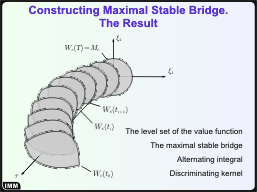So, the result of execution of the procedure is a collection of convex polygons approximating time sections of the maximal stable bridge.

Some synonyms for the term "maximal stable bridge" are given.

## Slide 8. The Idea of Constructing Singular Surfaces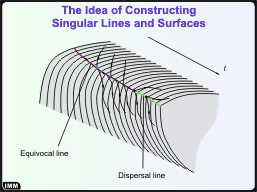The main idea of detecting singular points on the boundary of the level set of the vaule function (the maximal stable bridge) is the following. Often, the singularities are connected to some non-differentiabilities of the value function. These non-differentiabilities give non-smoothnesses on the boundary of level sets of the value function. So, the main object of detection is "sharp" points on the boundary of constructing approximating polygonal time sections.

So, after producing a new polygon, the algorithm searches for vertices of this polygon having the inner angle less than the straight angle minus some threshold value. These vertices are denoted as singular points. Then, the points are classified and joined into singular lines going along the boundary of the level set. Finally, singular lines taken from a collection of level sets give singular surfaces.

## Slide 9. The Idea of Singularities ClassificationThe classification of singular points is based on the dynamics of the cone of external normals to the section polygon at the singular point. At first, two singular points are counted as neighbor if their cones of external normals intersect.

After establishing the neighborhood of the points, their cones of external normals are compared. If in comparison with the cone of the previous point (in the backward time), both boundary vectors go outside the cone then the new point is marked as dispersal. If, vice versa, the boundaries go inside, the new point is universal (focal). And, finally, if the cone "rotates", that is, both boundaries go to the same direction, the new point is marked as equivocal.

If to go deeper into the ideology and to consider behavior of the optimal motions in the approximating discretized game, the classification coincides with the one given in the Isaacs' book.

## Slide 10. Problem of Aerial Interception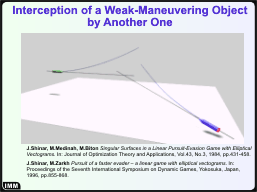As a test example, a problem of aerial interception of one weak-maneuvering object by another one is considered. This problem was studied analytically in details by Prof. J.Shinar and his colleagues (the main publications with their results are shown).

The matter of the problem is the following. There is a coming enemy missile (green), we launch an anti-missile (blue). The launch is such that there is exact collision along the nominal trajectories. Then during closing, the objects can slightly change their courses by means of their control accelerations orthogonal to the current velocity. Since the acceleration are relatively small, the objects are called weak-maneuvring.

The objective of the pursuer (the anti-missile) is to minimize the miss to the evaider (the missile).

## Slide 11. Formalization of the Problem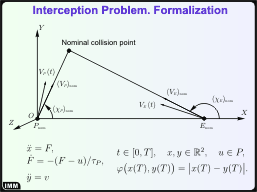A coordinate system is introduced in the following way. The origin is put to the pursuer. The axis OX coincides with the initial line-of-sight. The axis OY lays in the plane defined by the initial nominal velocities of the objects. The axis OZ completes the right-hand triple.

Since during the closing the velocities change slightly, it is possible to linearize the system along the nominal trajectories. After the linearization, the longitudinal motion becomes uniform and can be neglected. So, investigation of the initial non-linear three-dimentional motion can be changed by studying a linear two-dimensional motion of the objects in the lateral plane OYZ. Also, non-fixed instant of the minimal closing is changed by a fixed instant T of the nominal collision.

The dynamics of the objects are shown in the figure. The dynamics of the evaider is of the second order (the evaider controls its acceleration directly). The dynamics of the pursuer is of the third order because we take into account the inertiality of the servo-mechanisms. The quantity τP is the time constant describing the inertiality.

The payoff function is the lateral distance between the objects at the instant of the nominal collision. Of course, generally speaking, it differs from the true miss. But due to large velocities, the instant of the closest miss is near the instant of the nominal collision, and these two distances are approximately the same.

The constraints P for the pursuer's control and Q for the evaider's control are ellipses got after projecting circles of the control accelerations of the objects into the lateral plane. Eccentricities of the ellipses are defined by the angles of the nominal velocities and, generally speaking, are different.

## Slide 12. Dynamics in the Standard Form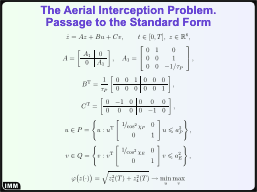Here, one can see the description of the game in the standard form.

## Slide 13. A Level Set of the Value Function for the Case of a Slower Pursuer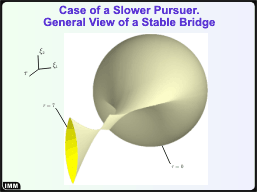It turns out that for any combination of parameters of the game there is a range of payoff values, when corresponding level sets of the value function has "narrow throat", that is, degeneration of their time sections to a point or almost to a point. With that, the structure of the set near the throat is sufficiently different for the cases of fast and slow pursuer (that is, for the cases (VP)nom < (VE)nom and (VP)nom > (VE)nom).

This slide shows a level set for the case of slower pursuer. The example is computed for the following data: τP = 1, T = 7.0, cos (χP)nom = 0.67, cos (χE)nom = 0.71, c = 1.546.

The circle at the right is the terminal set, that is, the level set of the payoff function corresponding to the value of the parameter c.

## Slide 14. A Large View of the Narrow Throat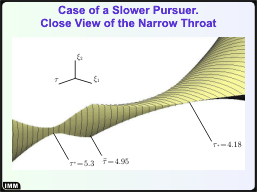Here, a large view of the narrow throat is given. Some important instants of the backward time τ are shown.

## Slide 15. Singularities in this ProblemThis slide contains the level set with the singular lines drawn on its boundary. The green-cian color denotes dispersal lines, magenta shows the equivocal lines, universal (focal) lines are given in blue.

## Slide 16. Singular Lines near the Narrow Throat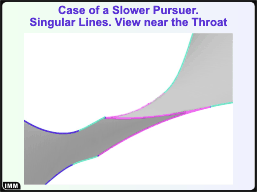A figure showing in details what is happening near the narrow throat.

## Slide 17. Singularities and Optimal Motions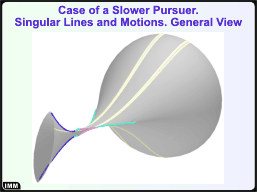Also, a program was worked out, which simulates developing a bundle of optimal motions on the basis of information about the singular lines. This program uses the fact that an optimal motion starting from a point laying on the boundary of a bridge generates a bundle of motions, all of which go along the boundary.

In this figure, one can see the previously shown picture added by a bundle of optimal motions emanated from a point belonging to the initial section of the bridge (on the left). The bundle is drawn by yellow lines. Separate motions of this bundle reach all singularities of this system.

## Slide 18. Singularities and Optimal Motions near the Narrow Throat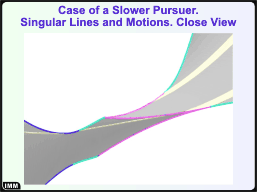Here, one can see an enlarged fragment of the previous picture, which shows the configuration near the narrow throat. The colors are the same: dispersal lines are green-cian, universal (focal) lines are blue, equivocalnesses are magenta, and the motions are yellow.

The initial motion comes from the right to the universal line and goes along it until the line becomes dispersal. At this point, the motion splits into two, one of which is seen, another one goes at the back side of the "comb". Each of these two motions reaches an equivocal line and then goes along it. With that, at every instant each of them generates a motion leaving the equivocalness and goes upstairs. Totality of all these "leaving" motions looks like two yellow strips going to the upper part of the tube. The motions, which go along the equivocal lines, reach the point, where the type changes to dispersal. At these points, each of these two motions again splits into two. One of the motions from the pair goes upstairs as a boundary line of the strips. Another one goes downstairs, what can be seen in the previous slide.

## Slide 19. L.S.Pontryagin's Test ExampleIn papers in 1960's, L.S.Pontryagin used a test example shown in the upper part of the slide as a test one to illustrate his theoretical methods. This example describes two independent inertial points in the plane influenced by dry friction. The aim of the first object (x-object) is to minimize the distance between objects at the terminal instant; the y-objects counteracts to this.

Further, this example was generalized by adding higher derivatives to the left-hand side of objects' descriptions (see the bottom of the slide).

## Slide 20. A Problem of Generalized Test Type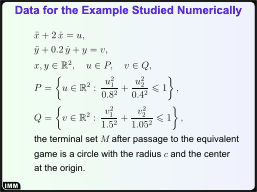Here, a problem of L.S.Pontryagin's Generalized Test Example is shown. Its peculiarity is that level sets of the value function for this problem also have narrow throats for some range of values.

## Slide 21. A Level Set for the Problem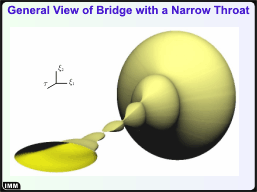Since the second controlled object is an oscillator with a friction, the set also has "pulsing" structure.

## Slide 22. The Narrow Throat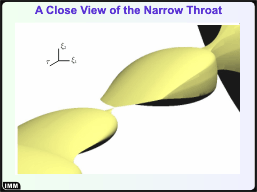Here, one can see enlarged part of the set with the most narrow place. Lines of non-smoothness on the boundary of the set say that there are some singular lines.

## Slide 23. Singularities and Optimal Motions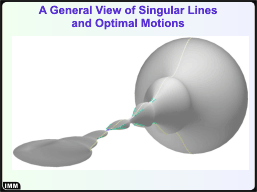Here, a general view of the bridge, sigularities, and some bundle of optimal motions are shown. In this case, the bundle is not so rich, now it consists of two motions only.

## Slide 24. Peculiarities of the Bundle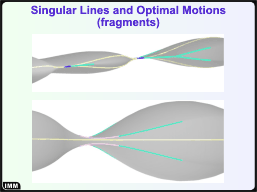This slide gives pictures of two peculiarities of the bundle. The first one (see the upper figure) is that the initial motion reaches the universal line, then goes along it and splits into two motions. But later, these two motions come together at the next universal line. The resulting motion finally splits near the throat, and two appeared motions go independently up to the terminal set.

Another thing about this bundle is that no motions from it reach equivocal lines (see the lower figure). This explains its poorness.

## Slide 25. Another Bundle of Optimal MotionsTo obtain a bundle, which is not so poor, another initial point was taken. A general view of the resulting bundle is given.

## Slide 26. Configuration of the Bundle near Equivocal Line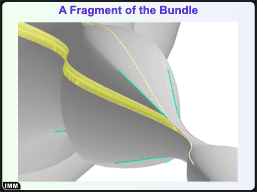An enlarged fragment is given here. The initial motion reaches the equivocal line, and one can see again a strip consisting of optimal motions.

## Slide 27. Conclusions